# 9) For the circuit shown in the figure, the switch S is initially open and the...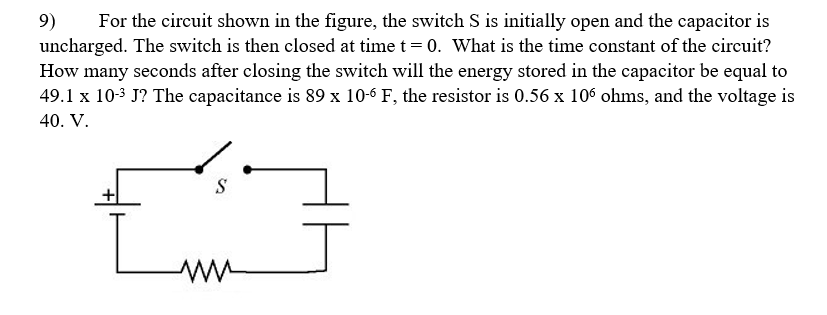9) For the circuit shown in the figure, the switch S is initially open and the capacitor is uncharged. The switch is then closed at time t 0. What is the time constant of the circuit? How many seconds after closing the switch will the energy stored in the capacitor be equal to 49.1 x 10-3 J? The capacitance is 89 x 10-6 F, the resistor is 0.56 x 106 ohms, and the voltage is 40. V

Given,

C = 89 x 10^-6 F ; R = 0.56 x 10^6 Ohms ; V = 40 V ; U = 49.1 x 10^-3 J

We know that, time constant is given by:

tau = RC

tau = 0.56 x 10^6 x 89 x 10^-6 = 49.84 s

Hence, tau = 49.84 s

the energy is:

U = 1/2 Q^2/C

Q = sqrt (2 U C)

Q = sqrt (2 x 49.1 x 10^-3 x 89 x 10^-6) = 0.0029 C

We know that,

Q = C V (1 - e^-t/RC)

0.0029 = 89 x 10^-6 x 40 (1 - e^-t/49.84)

0.81 = 1 - e^-t/49.84

e^-t/49.84 = 1 - 0.81 = 0.19

-t/49.84 = -1.66

t = 1.66 x 49.84 = 82.73 s

Hence, t = 82.73 s

#### Earn Coin

Coins can be redeemed for fabulous gifts.

Similar Homework Help Questions
• ### For the circuit shown in the figure, the switch S is initially open and the capacitor is...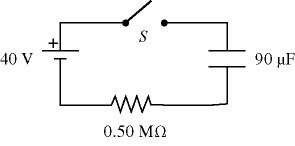For the circuit shown in the figure, the switch S is initially open and the capacitor is uncharged. The switch is then closed at time t = 0. How many seconds after closing the switch will the energy stored in the capacitor be equal to 50.2 mJ?

• ### For the circuit shown in the figure, the switch S is initially open and the capacitor...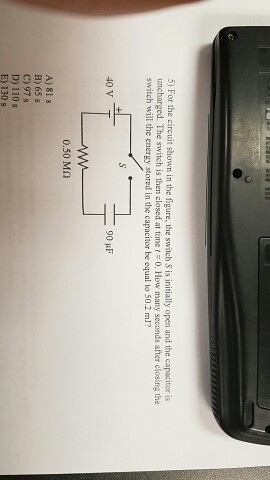For the circuit shown in the figure, the switch S is initially open and the capacitor is uncharged. The switch is then dosed at time t = 0. How many seconds after closing the switch will the energy stored in live capacitor be equal to 50.2 mJ?

• ### The switch is closed at time t = 0. The battery, shown in the figure has...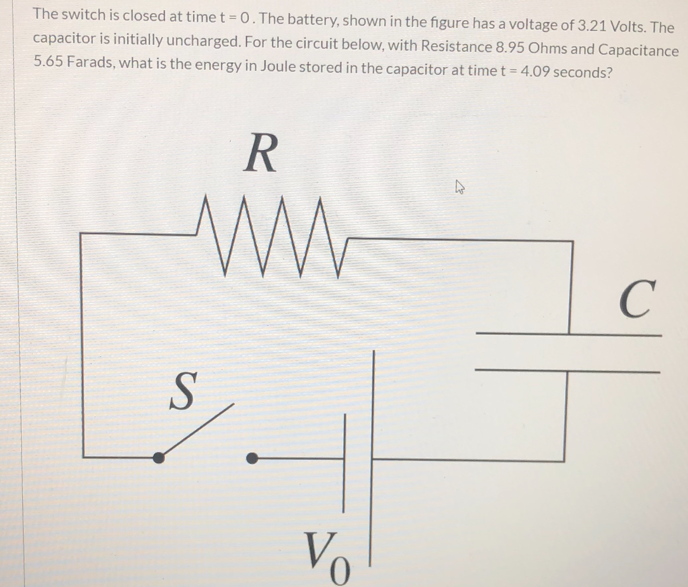The switch is closed at time t = 0. The battery, shown in the figure has a voltage of 3.21 Volts. The capacitor is initially uncharged. For the circuit below, with Resistance 8.95 Ohms and Capacitance 5.65 Farads, what is the energy in Joule stored in the capacitor at time t= 4.09 seconds?

• ### In the RC circuit shown, switch S is initially open and the capacitor uncharged. The switch...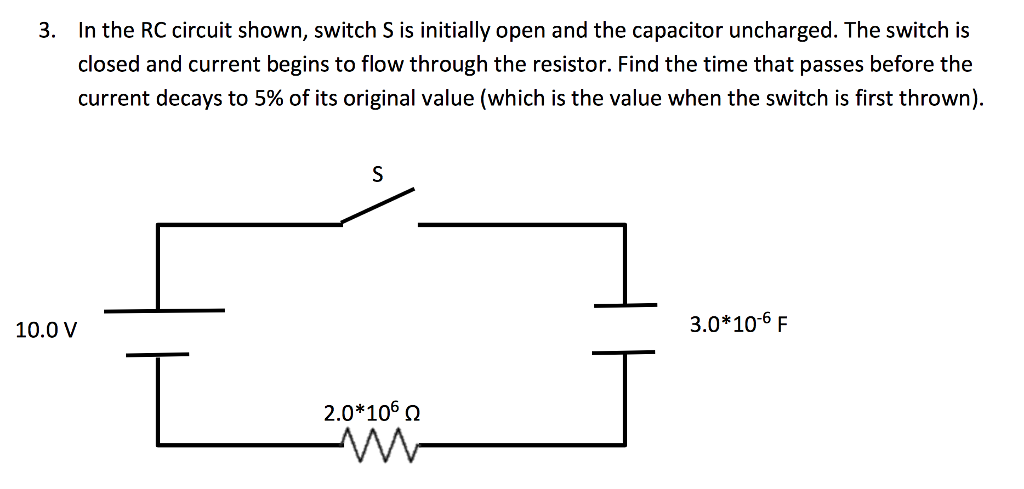In the RC circuit shown, switch S is initially open and the capacitor uncharged. The switch is closed and current begins to flow through the resistor. Find the time that passes before the current decays to 5% of its original value (which is the value when the switch is first thrown).

• ### Initially, for the circuit shown, the switch S is open and the capacitor voltage is 150...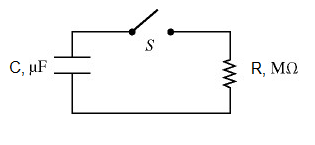Initially, for the circuit shown, the switch S is open and the capacitor voltage is 150 V. The resistance and capacitance are 1.2 M? and 34 µF respectively. The switch S is closed at time t = 0. In Figure, the charge in µC on the capacitor, when the current in the circuit is 15 µA, is:

• ### in the circuit the switch is initially open as shown w 1) In the circuit, the...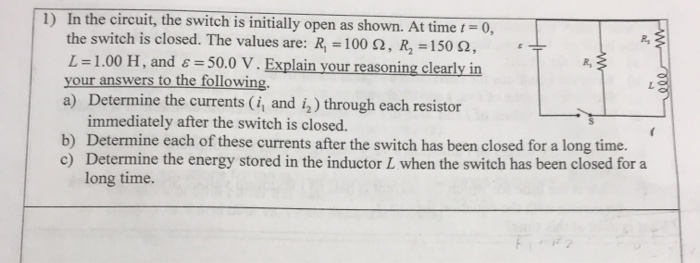in the circuit the switch is initially open as shown w 1) In the circuit, the switch is initially open as shown. At time 0 , the switch is closed. The values are: R = 100 92, R = 150 2, L=1.00 H, and 8 = 50.0 V. Explain your reasoning clearly in your answers to the following. a) Determine the currents (i, and in through each resistor immediately after the switch is closed. b) Determine each of these currents...

• ### i need 4e and 5b 4. For the circuit shown, the switch is initially open and...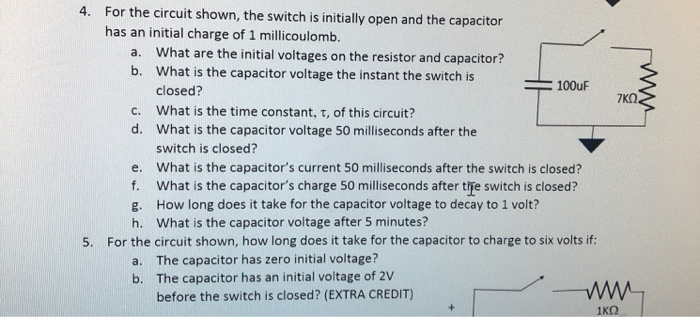i need 4e and 5b 4. For the circuit shown, the switch is initially open and the capacitor has an initial charge of 1 millicoulomb. a. What are the initial voltages on the resistor and capacitor? b. What is the capacitor voltage the instant the switch is 100uF closed? 7ΚΩ. c. What is the time constant, t, of this circuit? d. What is the capacitor voltage 50 milliseconds after the switch is closed? e. What is the capacitor's current 50...

• ### In the circuit shown, the capacitor is initially uncharged. At time t = 0, switch S...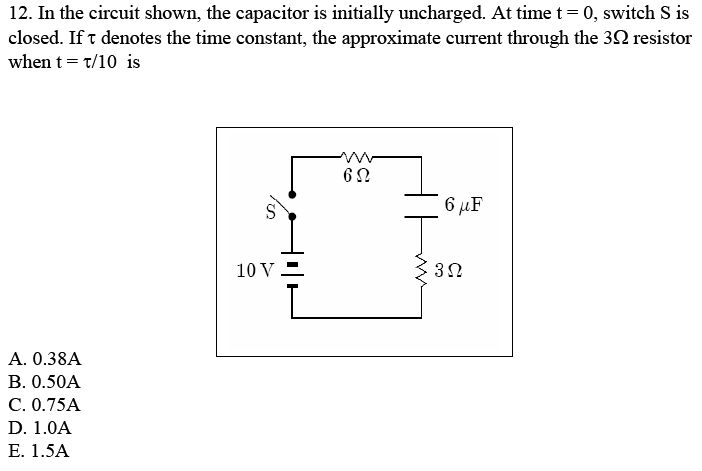In the circuit shown, the capacitor is initially uncharged. At time t = 0, switch S is closed. If tau denotes the time constant, the approximate current through the 3 Ohm resistor when t = tau/10 is 0.38A 0.50A 0.75A 1.0A 1.5A

• ### Consider the RC circuit in the figure. The switch has been open for a long time...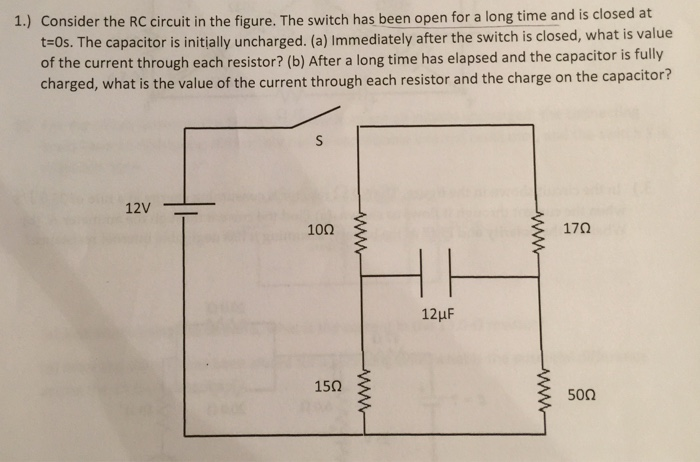Consider the RC circuit in the figure. The switch has been open for a long time and is closed at t=0s. The capacitor initially uncharged. (a) Immediately after the switch is closed, what is value of the current through each resistor? (b) After a long time has elapsed and the capacitor is fully charged, what is the value of the current through each resistor and the charge on the capacitor?